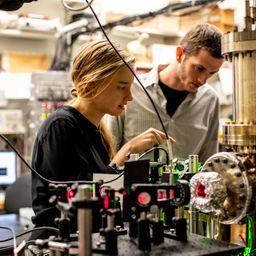## 120 \mathrm{V} \text { and } 1.2 \times 10^{7} \mathrm{V}

Quantum Physics

Atomic Physics

### Discussion

You must be signed in to discuss.
##### Top Physics 103 Educators##### Christina K.

Rutgers, The State University of New Jersey##### Andy C.

University of Michigan - Ann Arbor

LB

### Video Transcript

Okay, So for this question, um, we know that the extremes of the x ray range or given and we want to get the, um accelerating voltage to, uh, produce wavelength that these two extremes. So we know that Lambda is equal to, let's say, the 1st 11 times 10 to the minus eight meters, one times, 10 to the minus eight meters. And then we need accelerating voltages to produce wavelengths of those two extremes. Um, so I think that what we want to do is, uh, set the energy associated with this equal to the this faux time with energy of the, um, like, equal to the energy due to a voltage. So be kind of like, assuming that the work function of some metal goes to zero like we're gonna use the general photo electric formula says the energy of the foe time minus the work function. And then we'll set this equal to zero. And then kinetic energy, um, is equal to e times for you. So, um so solving pervy we got V is equal to one over e times e and then e is equal to HC, divided by lambda. So if I go ahead and plug in these numbers. Let's see what I got. So collects constant speed of light. Um, over easy. And I got 124 bolts for the lower extreme and for the higher extreme. So I'll say that B one and then be to this Gonna be Lambda one hoops now, just kind of looks like a big lambda. There we go. And then Lambda Thio, I would say, Let's see, That's one time the minus 13 repeating the calculation. Um, I got this quite a large voltage. Lt. 12345671.24 times 10 of the seven. I guess I should just use to sink things. Is that all we're given? Yeah, 1.2 times. 10 to the seven and then this one. I suppose it should also just be 100 and 20. So I'll go ahead and fix that. I try to find these sick, big errors when I can. Um, but of course, you can always just repeat the calculations. Um, keeping all the sig figsUniversity of Washington

#### Topics

Quantum Physics

Atomic Physics

##### Top Physics 103 Educators##### Christina K.

Rutgers, The State University of New Jersey##### Andy C.

University of Michigan - Ann Arbor

LB